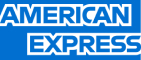New update is available. Click here to update.
Topics

# Check If One String Is A Rotation Of Another String

Moderate0/80
Average time to solve is 15m+9 more companies

## Problem statement

You are given two Strings 'P' and 'Q' of equal length.

Your task is to return 1 if String 'P' can be converted into String 'Q' by cyclically rotating it to the right any number of times ( Possibly Zero ), else return 0.

A cyclic rotation to the right on String 'A' consists of taking String 'A' and moving the rightmost character to the leftmost position. For example, if 'A' = "pqrst", then it will be "tpqrs" after one cyclic rotation on 'A'.

For example:
``````Consider the two strings 'P' = "abfyg" and 'Q' = "gabfy"

If we cyclically rotate String 'P' to the right once. The resulting string P becomes "gabfy" which is equal to String 'Q'.

Therefore it is possible to convert String 'P' to String 'Q'.
``````
Detailed explanation ( Input/output format, Notes, Images )
Sample Input 1:
``````abac
baca
``````
Sample Output 1:
``````1
``````
Explanation For Sample Input 1:
``````Let's try rotating the String 'P' to the right and try all the possible rotations:

1) If we rotate "abac" to the right, the resulting string becomes "caba".

2) If we rotate "caba" to the right, the resulting string becomes "acab".

3) If we rotate "acab" to the right, the resulting string becomes "baca" which is equal to String 'Q'.

Therefore it is possible to convert String 'P' to String 'Q'. Hence, we will return 1 in this case.
``````
Sample Input 2:
``````aabca
bacaa
``````
Sample Output 2:
``````0
``````
Constraints:
``````1 <= |P| , |Q| <= 10^5
|P| = |Q|

String 'P' and 'Q' have the same length and contain only lowercase English letters.

Time Limit: 1 sec
``````
``````Can you solve this in O(N) time?